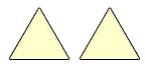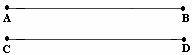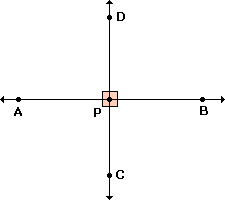Definition Of Congruent

Congruent means having the same measure.

Congruent angles are angles that have the same measure.
An equilateral triangle has all its angles measuring 60°. So, the angles of an equilateral triangle are congruent.
A square and a rectangle have all their angles measuring 90°. So, the angles of a square and a rectangle are congruent.
Congruent Figures have the same shape and size.Congruent Segments have the same length.Solved Example on Congruent

Ques: Line CD bisects another line AB at point P. Name any two congruent angles formed.

Choices:

A. Angles APD and CPD
B. Angles CPB and APD
C. Angles APB and APD
D. Angles CPB and CPD